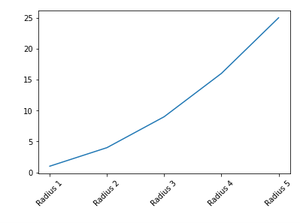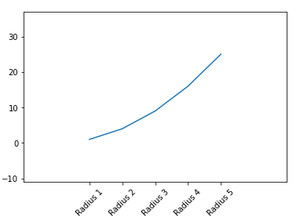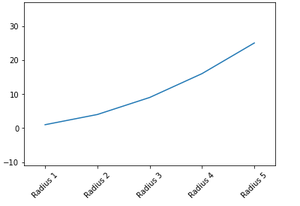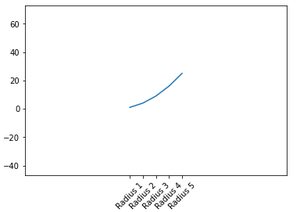# Matplotlib.pyplot.margins() function in Python

• Difficulty Level : Expert
• Last Updated : 03 Jan, 2021

Prerequisites: Matplotlib

Matplotlib.pyplot.margins() is a function used to set the margins of the x and y axes. All input parameters must be a float that too within the range [0, 1]. We cannot pass both positional and keyword arguments at once as that will raise a TypeError. The padding added to each limit of the axes is the margin times the data interval for that axis. If no arguments are provided, the existing margins remain in place. Specifying margins changes auto-scaling.

Attention geek! Strengthen your foundations with the Python Programming Foundation Course and learn the basics.

To begin with, your interview preparations Enhance your Data Structures concepts with the Python DS Course. And to begin with your Machine Learning Journey, join the Machine Learning - Basic Level Course

• If only one float value if provided, that is taken as margins for both x and y axes.
• If two float values are provided, they will be taken to specify x-margin and y-margin axes respectively.

Syntax:

margins(x, y, tight)

Parameters

x, y : Used to specify margin values for x and y axes. These can only be used individually.

tight : Boolean

• If this parameter is specified as True, then it is considered the specified margins require no additional padding to match tick marks.
• If this parameter is set to None, it will preserve original setting.

Various implementation for using this function is given below:

Example: General application

## Python3

 `import` `matplotlib.pyplot as plt`` ` `x ``=` `[``1``, ``2``, ``3``, ``4``, ``5``]``labels ``=` `[``'Radius 1'``, ``'Radius 2'``, ``'Radius 3'``, ``'Radius 4'``, ``'Radius 5'``]``y ``=` `[i``*``*``2` `for` `i ``in` `x]`` ` `plt.plot(x, y)``plt.xticks(x, labels, rotation``=``45``)``plt.margins()``plt.show()`

Output:Example : Passing arguments to the margins() function

## Python3

 `import` `matplotlib.pyplot as plt`` ` `x ``=` `[``1``, ``2``, ``3``, ``4``, ``5``]``labels ``=` `[``'Radius 1'``, ``'Radius 2'``, ``'Radius 3'``, ``'Radius 4'``, ``'Radius 5'``]``y ``=` `[i``*``*``2` `for` `i ``in` `x]`` ` `plt.plot(x, y)``plt.xticks(x, labels, rotation``=``45``)``plt.margins(``0.5``)``plt.show()`

Output:Example: Passing different values for x and y that are less than 1, the graph produced will be zoomed in

## Python3

 `import` `matplotlib.pyplot as plt`` ` `x ``=` `[``1``, ``2``, ``3``, ``4``, ``5``]``labels ``=` `[``'Radius 1'``, ``'Radius 2'``, ``'Radius 3'``, ``'Radius 4'``, ``'Radius 5'``]``y ``=` `[i``*``*``2` `for` `i ``in` `x]`` ` `plt.plot(x, y)``plt.xticks(x, labels, rotation``=``45``)``plt.margins(x``=``0.1``, y``=``0.5``)``plt.show()`

Output:Example: Passing different values for x and y that are greater than , the graph produced will be zoomed out

## Python3

 `import` `matplotlib.pyplot as plt`` ` `x ``=` `[``1``, ``2``, ``3``, ``4``, ``5``]``labels ``=` `[``'Radius 1'``, ``'Radius 2'``, ``'Radius 3'``, ``'Radius 4'``, ``'Radius 5'``]``y ``=` `[i``*``*``2` `for` `i ``in` `x]`` ` `plt.plot(x, y)``plt.xticks(x, labels, rotation``=``45``)``plt.margins(x``=``2``, y``=``2``)``plt.show()`

Output:My Personal Notes arrow_drop_up